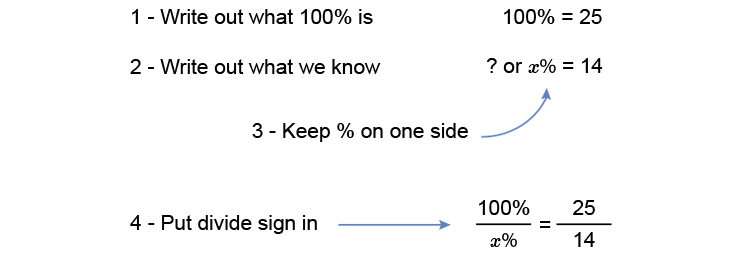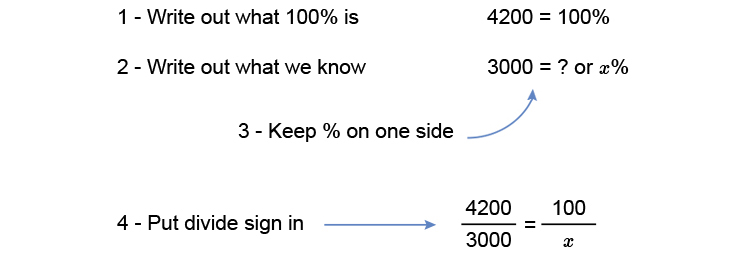# Finding a percentage

Example 1

14 People out of 25 were female. What percentage were female?Multiply both sides by x to get x on its own

(100timescancelx)/cancelx=(25timesx)/14

100=(25x)/14

Multiply both sides by 14 to get x on its own

100times14=(25xtimescancel14)/cancel14

100times14=25x

Divide both sides by 25 to get x on its own

(4\ \ cancel100times14)/(1\ \ cancel25)=(cancel25x)/cancel25

4times14=x

x=56

Answer: 56% were female.

Example 2

What percentage of 4200 is 3000?Multiply both sides by x to get x on its own (what ever you do to one side you do to the other).

(xtimes4200)/3000=(100timescancelx)/cancelx

Multiply both sides by 3000 to get x on its own

(cancel3000times4200x)/cancel3000=100times3000

4200x=100times3000

Divide both sides by 4200 to get x on its own

(cancel4200x)/cancel4200=(100times3000)/4200

x=(100times30cancel00)/(42cancel00)

x=(100timescancel30)/cancel42\ \ \ 15/21

x=1500/21

(Working out on a calculator)

x=71.43

Answer: 3000 is 71.43% of 4200